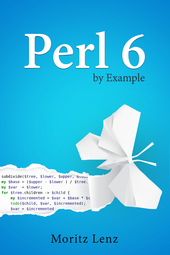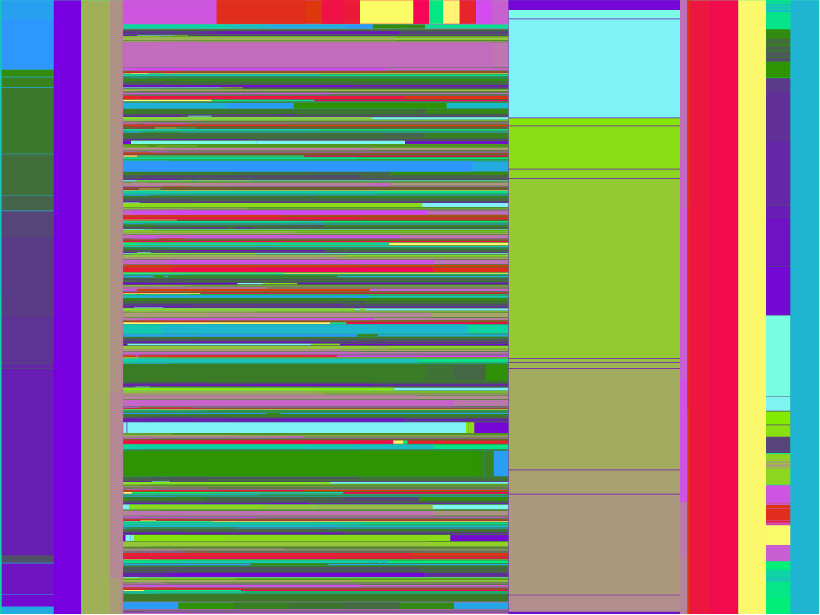# Perl 6 By Example: Functional Refactorings for Directory Visualization CodeThis blog post is part of my ongoing project to write a book about Perl 6.

If you're interested, either in this book project or any other Perl 6 book news, please sign up for the mailing list at the bottom of the article, or here. It will be low volume (less than an email per month, on average).

In the last installment we've seen some code that generated tree maps and flame graphs from a tree of directory and file sizes.

There's a pattern that occurs three times in that code: dividing an area based on the size of the files and directories in the tree associated with the area.

Extracting such common code into a function is a good idea, but it's slightly hindered by the fact that there is custom code inside the loop that's part of the common code. Functional programming offers a solution: Put the custom code inside a separate function and have the common code call it.

Applying this technique to the tree graph flame graph looks like this:

``````sub subdivide(\$tree, \$lower, \$upper, &todo) {
my \$base = (\$upper - \$lower ) / \$tree.total-size;
my \$var  = \$lower;
for \$tree.children -> \$child {
my \$incremented = \$var + \$base * \$child.total-size;
todo(\$child, \$var, \$incremented);
\$var = \$incremented,
}
}

sub flame-graph(\$tree, :\$x1!, :\$x2!, :\$y!, :\$height!) {
return if \$y >= \$height;
take 'rect' => [
x      => \$x1,
y      => \$y,
width  => \$x2 - \$x1,
height => 15,
style  => "fill:" ~ random-color(),
title  => [\$tree.name ~ ', ' ~ format-size(\$tree.total-size)],
];
return if \$tree ~~ File;
subdivide( \$tree, \$x1, \$x2, -> \$child, \$x1, \$x2 {
flame-graph( \$child, :\$x1, :\$x2, :y(\$y + 15), :\$height );
});
}

sub tree-map(\$tree, :\$x1!, :\$x2!, :\$y1!, :\$y2) {
return if (\$x2 - \$x1) * (\$y2 - \$y1) < 20;
take 'rect' => [
x      => \$x1,
y      => \$y1,
width  => \$x2 - \$x1,
height => \$y2 - \$y1,
style  => "fill:" ~ random-color(),
title  => [\$tree.name],
];
return if \$tree ~~ File;

if \$x2 - \$x1 > \$y2 - \$y1 {
# split along the x-axis
subdivide \$tree, \$x1, \$x2, -> \$child, \$x1, \$x2 {
tree-map \$child, :\$x1, :\$x2, :\$y1, :\$y2;
}
}
else {
# split along the y-axis
subdivide \$tree, \$y1, \$y2, -> \$child, \$y1, \$y2 {
tree-map \$child, :\$x1, :\$x2, :\$y1, :\$y2;
}
}
}
``````

The newly introduced subroutine `subdivide` takes a directory tree, a start point and an end point, and finally a code object `&todo`. For each child of the directory tree it calculates the new coordinates and then calls the `&todo` function.

The usage in subroutine `flame-graph` looks like this:

``````subdivide( \$tree, \$x1, \$x2, -> \$child, \$x1, \$x2 {
flame-graph( \$child, :\$x1, :\$x2, :y(\$y + 15), :\$height );
});
``````

The code object being passed to `subdivide` starts with `->`, which introduces the signature of a block. The code block recurses into `flame-graph`, adding some extra arguments, and turning two positional arguments into named arguments along the way.

This refactoring shortened the code and made it overall more pleasant to work with. But there's still quite a bit of duplication between `tree-map` and `flame-graph`: both have an initial termination condition, a `take` of a rectangle, and then a call or two to `subdivide`. If we're willing to put all the small differences into small, separate functions, we can unify it further.

If we pass all those new functions as arguments to each call, we create an unpleasantly long argument list. Instead, we can use those functions to generate the previous functions `flame-graph` and `tree-map`:

``````sub svg-tree-gen(:&terminate!, :&base-height!, :&subdivide-x!, :&other!) {
sub inner(\$tree, :\$x1!, :\$x2!, :\$y1!, :\$y2!) {
return if terminate(:\$x1, :\$x2, :\$y1, :\$y2);
take 'rect' => [
x      => \$x1,
y      => \$y1,
width  => \$x2 - \$x1,
height => base-height(:\$y1, :\$y2),
style  => "fill:" ~ random-color(),
title  => [\$tree.name ~ ', ' ~ format-size(\$tree.total-size)],
];
return if \$tree ~~ File;
if subdivide-x(:\$x1, :\$y1, :\$x2, :\$y2) {
# split along the x-axis
subdivide \$tree, \$x1, \$x2, -> \$child, \$x1, \$x2 {
inner(\$child, :\$x1, :\$x2, :y1(other(\$y1)), :\$y2);
}
}
else {
# split along the y-axis
subdivide \$tree, \$y1, \$y2, -> \$child, \$y1, \$y2 {
inner(\$child, :x1(other(\$x1)), :\$x2, :\$y1, :\$y2);
}
}
}
}

my &flame-graph = svg-tree-gen
terminate   => -> :\$y1, :\$y2, | { \$y1 > \$y2 },
base-height => -> | { 15 },
subdivide-x => -> | { True },
other       => -> \$y1 { \$y1 + 15 },

my &tree-map = svg-tree-gen
terminate   => -> :\$x1, :\$y1, :\$x2, :\$y2 { (\$x2 - \$x1) * (\$y2 - \$y1) < 20 },
base-height => -> :\$y1, :\$y2 {  \$y2 - \$y1 },
subdivide-x => -> :\$x1, :\$x2, :\$y1, :\$y2 { \$x2 - \$x1 > \$y2 - \$y1 },
other       => -> \$a { \$a },
;
``````

So there's a new function `svg-tree-gen`, which returns a function. The behavior of the returned function depends on the four small functions that `svg-tree-gen` receives as arguments.

The first argument, `terminate`, determines under what condition the inner function should terminate early. For `tree-map` that's when the area is below 20 pixels, for `flame-graph` when the current y-coordinate `\$y1` exceeds the height of the whole image, which is stored in `\$y2`. `svg-tree-gen` always calls this function with the four named arguments `x1`, `x2`, `y1` and `y2`, so the `terminate` function must ignore the `x1` and `x2` values. It does this by adding `|` as a parameter, which is an anonymous capture. Such a parameter can bind arbitrary positional and named arguments, and since it's an anonymous parameter, it discards all the values.

The second configuration function, `base-height`, determines the height of the rectangle in the base case. For `flame-graph` it's a constant, so the configuration function must discard all arguments, again with a `|`. For `tree-graph`, it must return the difference between `\$y2` and `\$y1`, as before the refactoring.

The third function determines when to subdivide along the x-axis. Flame graphs always divide along the x-axis, so `-> | { True }` accomplishes that. Our simplistic approach to tree graphs divides along the longer axis, so only along the x-axis if `\$x2 - \$x1 > \$y2 - \$y1`.

The fourth and final function we pass to `svg-tree-gen` calculates the coordinate of the axis that isn't being subdivided. In the case of `flame-graph` that's increasing over the previous value by the height of the bars, and for `tree-map` it's the unchanged coordinate, so we pass the identity function `-> \$a { \$a }`.

The inner function only needs a name because we need to call it from itself recursively; otherwise an anonymous function ```sub (\$tree, :\$x1!, :\$x2!, :\$y1!, :\$y2!) { ... }``` would have worked fine.

Now that we have very compact definitions of `flame-graph` and `tree-map`, it's a good time to play with some of the parameters. For example we can introduce a bit of margin in the flame graph by having the increment in `other` greater than the bar height in `base-height`:

``````my &flame-graph = svg-tree-gen
base-height => -> | { 15 },
other       => -> \$y1 { \$y1 + 16 },
# rest as before
``````

Another knob to turn is to change the color generation to something more deterministic, and make it configurable from the outside:

``````sub svg-tree-gen(:&terminate!, :&base-height!, :&subdivide-x!, :&other!,
:&color=&random-color) {
sub inner(\$tree, :\$x1!, :\$x2!, :\$y1!, :\$y2!) {
return if terminate(:\$x1, :\$x2, :\$y1, :\$y2);
take 'rect' => [
x      => \$x1,
y      => \$y1,
width  => \$x2 - \$x1,
height => base-height(:\$y1, :\$y2),
style  => "fill:" ~ color(:\$x1, :\$x2, :\$y1, :\$y2),
title  => [\$tree.name ~ ', ' ~ format-size(\$tree.total-size)],
];
# rest as before
}
``````

We can, for example, keep state within the color generator and return a slightly different color during each iteration:

``````sub color-range(|) {
state (\$r, \$g, \$b) = (0, 240, 120);
\$r = (\$r + 5) % 256;
\$g = (\$g + 10) % 256;
\$b = (\$b + 15) % 256;
return "rgb(\$r,\$g,\$b)";
}
``````

`state` variables keep their values between calls to the same subroutine and their initialization runs only on the first call. So this function slightly increases the lightness in each color channel for each invocation, except when it reaches 256, where the modulo operator `%` resets it back to a small value.

If we plug this into our functions by passing `color => &color-range` to the calls to `svg-tree-gen`, we get much less chaotic looking output:And the flame graph:## More Language Support for Functional Programming

As you've seen in the examples above, functional programming typically involves writing lots of small functions. Perl 6 has some language features that make it very easy to write such small functions.

A common task is to write a function that calls a particular method on its argument, as we've seen here:

``````method total-size() {
\$!total-size //= \$.size + @.children.map({.total-size}).sum;
#                                        ^^^^^^^^^^^^^
}
``````

This can be abbreviated to `*.total-size`:

``````method total-size() {
\$!total-size //= \$.size + @.children.map(*.total-size).sum;
}
``````

This works for chains of method calls too, so you could write `@.children.map(*.total-size.round)` if `total-size` returned a fractional number and you wanted to the call `.round` method on the result.

There are more cases where you can replace an expression with the "Whatever" star `*` to create a small function. To create a function that adds 15 to its argument, you can write `* + 15` instead of `-> \$a { \$a + 15 }`.

If you need to write a function to just call another function, but pass more arguments to the second function, you can use the method assuming. For example ```-> \$x { f(42, \$x }``` can be replaced with `&f.assuming(42)`. This works also for named arguments, so `-> \$x { f(\$x, height => 42 ) }` can be replaced with `&f.assuming(height => 42)`.

## Summary

Functional programming offers techniques for extracting common logic into separate functions. The desired differences in behavior can be encoded in more functions that you pass in as arguments to other functions.

Perl 6 supports functional programming by making functions first class, so you can pass them around as ordinary objects. It also offers closures (access to outer lexical variables from functions), and various shortcuts that make it more pleasant to write short functions.

## Subscribe to the Perl 6 book mailing list

* indicates required